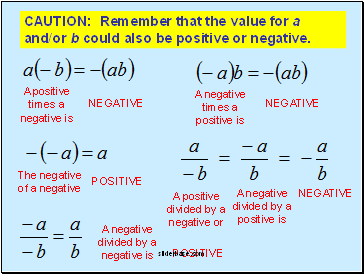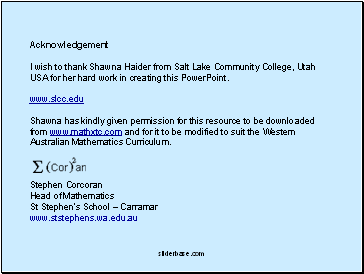# Real NumbersPage 2

#### WATCH ALL SLIDES

When multiplying, you can “associate” and multiply any factors first and then multiply the other factor.

The operations of both addition and multiplication are associative

Slide 10## Distributive property

The operation of multiplication distributes over addition

The distributive property also holds for a factor that is multiplied on the left.

Slide 11Rules of Signs

A positive times a negative is

NEGATIVE

A negative times a positive is

NEGATIVE

The negative of a negative

POSITIVE

CAUTION: Remember that the value for a and/or b could also be positive or negative.

A positive divided by a negative or

A negative divided by a positive is

NEGATIVE

A negative divided by a negative is

POSITIVE

Slide 12Acknowledgement

I wish to thank Shawna Haider from Salt Lake Community College, Utah USA for her hard work in creating this PowerPoint.

www.slcc.edu

Shawna has kindly given permission for this resource to be downloaded from www.mathxtc.com and for it to be modified to suit the Western Australian Mathematics Curriculum.

Stephen Corcoran